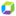cancel
Showing results for
Show  only  | Search instead for
Did you mean:

## Cumulative Sum - DQLDynatrace Enthusiast

Is it possible to plot a cumulative sum in DQL?

Let's take for example a graph that shows the count of a metric for the given time. How could we add a second line in the graph, that shows the cumulative sum of the previous metric?

For the first metric (M1) we would see, for each data point a value (M1V1). On the other hand, for the cumulative metric (M2), we would see a sum of all the values previous to that data point.

M2V5 = M1V1 + ... + M1V4

Would this be possible? Thank you in advance

0 REPLIES 0Featured Posts